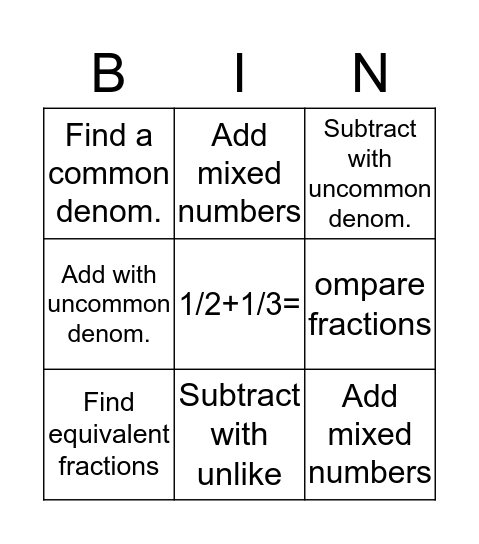# Math Fraction BingoThis bingo card has a free space and 24 words: Addition with common denom., Add with uncommon denom., Subtract with unlike, Find common denom, Add mixed numbers, Find a common denom., ompare fractions, Add mixed numbers, Subtract mixed numbers, Compare fractions, Subtract with uncommon denom, Add with common denom., Find equivalent fractions, Find common denom., Find equivalent fractions, Find equivalent fractions, Subtract with common denom., Subtract with uncommon denom., Equivalent fractions, Add mixed numbers, Add with uncommon denom., ompare fractions, Add with uncommon denom. and Subtract mixed numbers.

⚠ This card has duplicate items: Add with uncommon denom. (3), Add mixed numbers (3), ompare fractions (2), Subtract mixed numbers (2), Find equivalent fractions (3)

## Play Online

Share this URL with your players:For more control of your online game, create a clone of this card first.

## Probabilities

With players vying for a you'll have to call about __ items before someone wins. There's a __% chance that a lucky player would win after calling __ items.

Tip: If you want your game to last longer (on average), add more unique words/images to it.